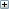# Altova StyleVision 2020 Professional Edition

 Auto-Calculations Based on Updated Nodes Scroll Home Prev Top Next More

If you wish to create an Auto-Calculation (second Auto-Calculation) that uses a node updated by another Auto-Calculation (first Auto-Calculation), there are two possible situations:

The two Auto-Calculations are in the same template.

The two Auto-Calculations are in different templates.

Auto-Calculations in the same template

When two Auto-Calculations are in the same template, the SPS applies the following procedure:

1.A node used in the XPath expression of the first Auto-Calculation is modified.

2.All node values in the XML document are read and all Auto-Calculations are executed.

3.Assuming that the first Auto-Calculation is executed correctly, it updates the specified XML node (call it Node-A). The second Auto-Calculation, which is based on Node-A, will be executed but will use the value of Node-A before Node-A was updated. This is because the value of Node-A was read before it was updated, and has not been read since then.

4.If the document is now edited in any way or if document views are changed (from and to Authentic View), then the values of nodes are read afresh and Auto-Calculations are executed.

5.The second Auto-Calculation is now carried out. (If this Auto-Calculation is intended to update a node, then, as is usual for node updates, a node used in the XPath expression will have to be changed before the update takes place.)

The time lag between the updating of Node-A and the evaluation of the second Auto-Calculation with the updated value of Node-A could be confusing for the Authentic View user. To ensure that this situation does not occur, it is best that the XPath expression of the second Auto-Calculation contain the XPath expression of the first Auto-Calculation—not the updated node itself. As a result, the second Auto-Calculation will execute with the input to the first Auto-Calculation and perform that Auto-calculation as part of its own Auto-Calculation. This enables it to be evaluated independently of the contents of Node-A.

Example

The first Auto-Calculation calculates the VAT amount of a product using the nodes for (i) the net price, and (ii) the VAT rate; it updates the VAT-amount node. The second Auto-Calculation calculates the gross price, which is the sum of net price and VAT amount; it updates the gross price node.

The Auto-Calculation to calculate the VAT amount is: NetPrice * VATRate div 100. When the VAT rate of the product is entered, the Auto-Calculation is executed and updates the VATAmount node.

If the Auto-Calculation to calculate the gross price is: NetPrice + VATAmount, then the Auto-Calculation will execute with the value of VATAmount that was read in before VATAmount was updated.

If, however, the Auto-Calculation to calculate the gross price is: NetPrice + (NetPrice * VATRate div 100), then the Auto-Calculation will execute with the value of VATRate and will update the GrossPrice node. The updated VatAmount node has been left out of the second Auto-Calculation.

For a detailed example, see

Auto-Calculations in different templates

When two Auto-Calculations are in different templates, a node updated by the first Auto-Calculation can be used by the second Auto-Calculation. This is because Auto-Calculations are calculated and nodes updated for each template separately. For an example of how this would work, seeSee also